Checking points collinear or not

Chapter 7 Class 10 Coordinate Geometry
Concept wise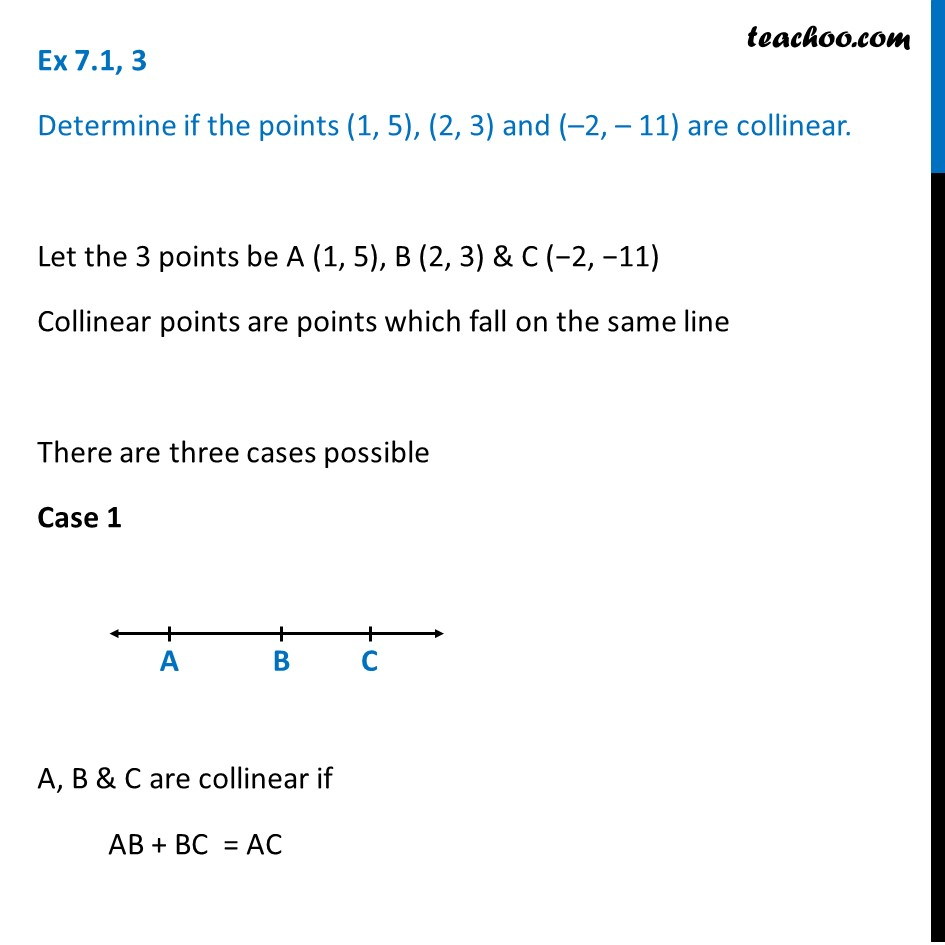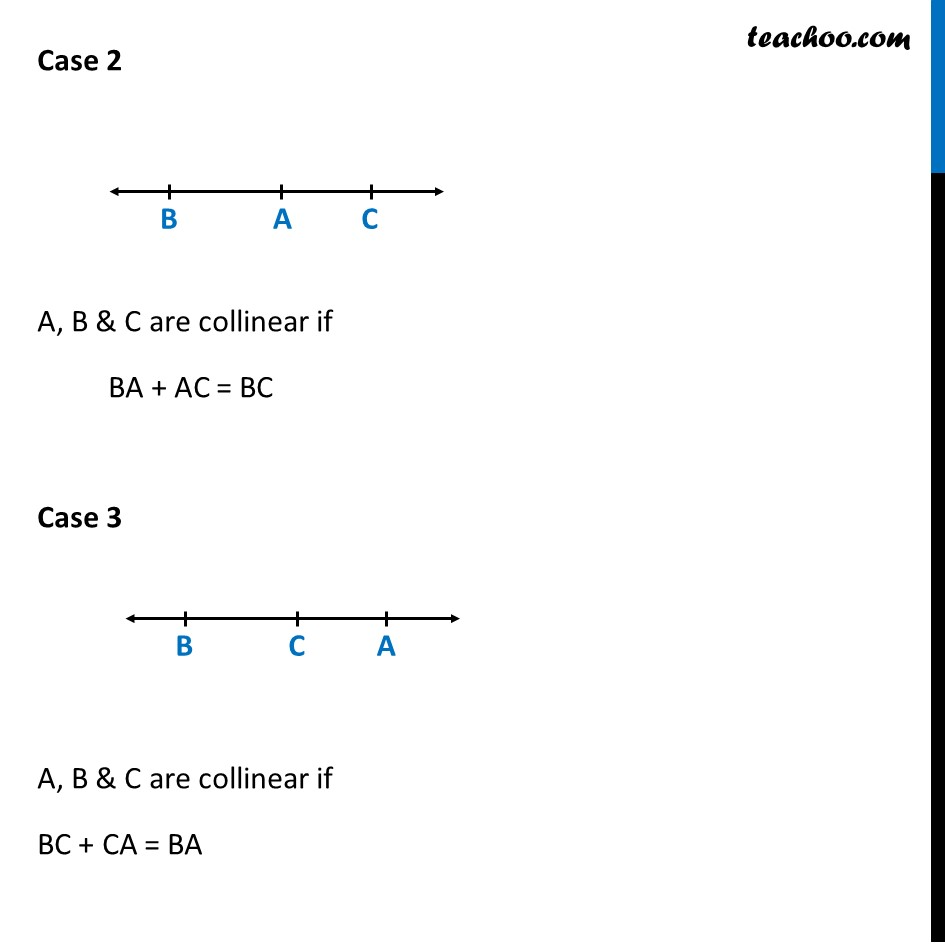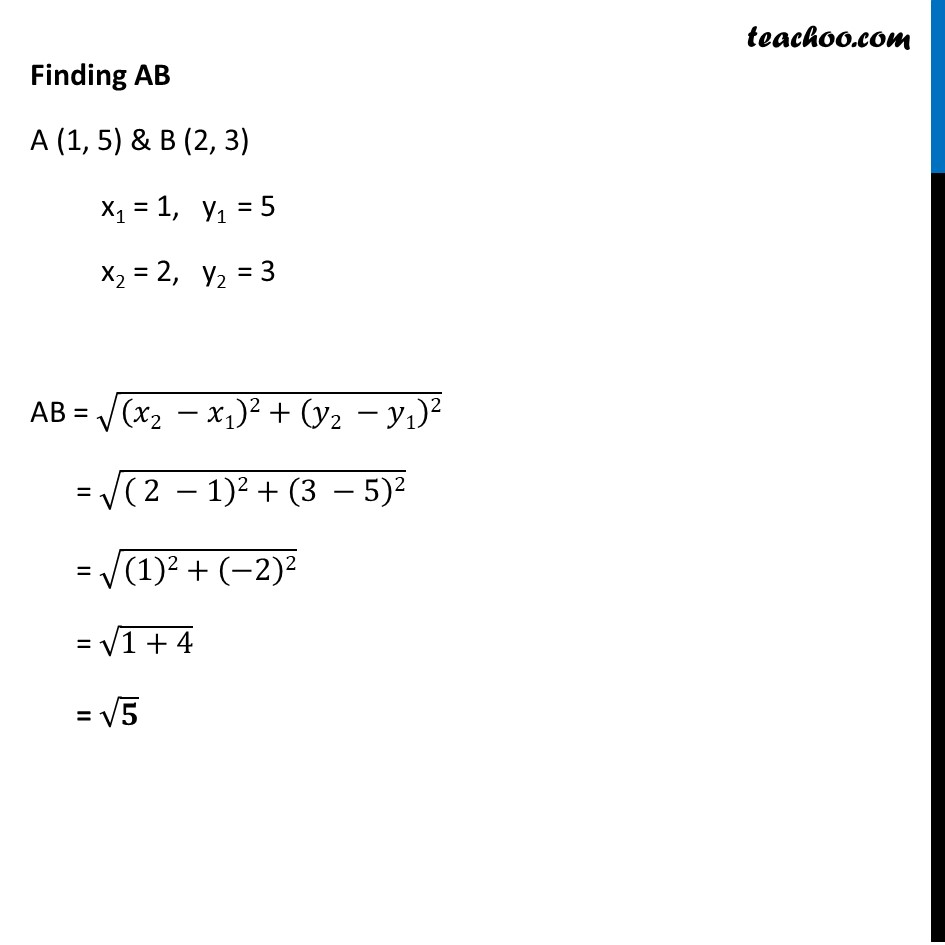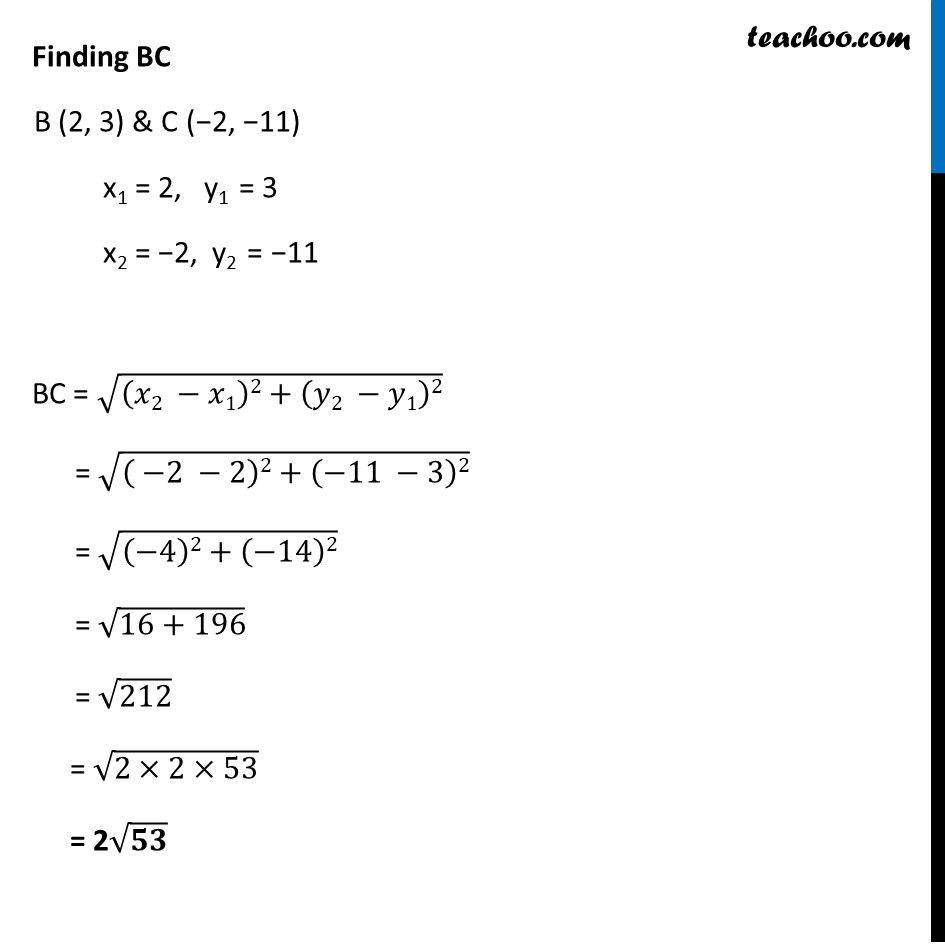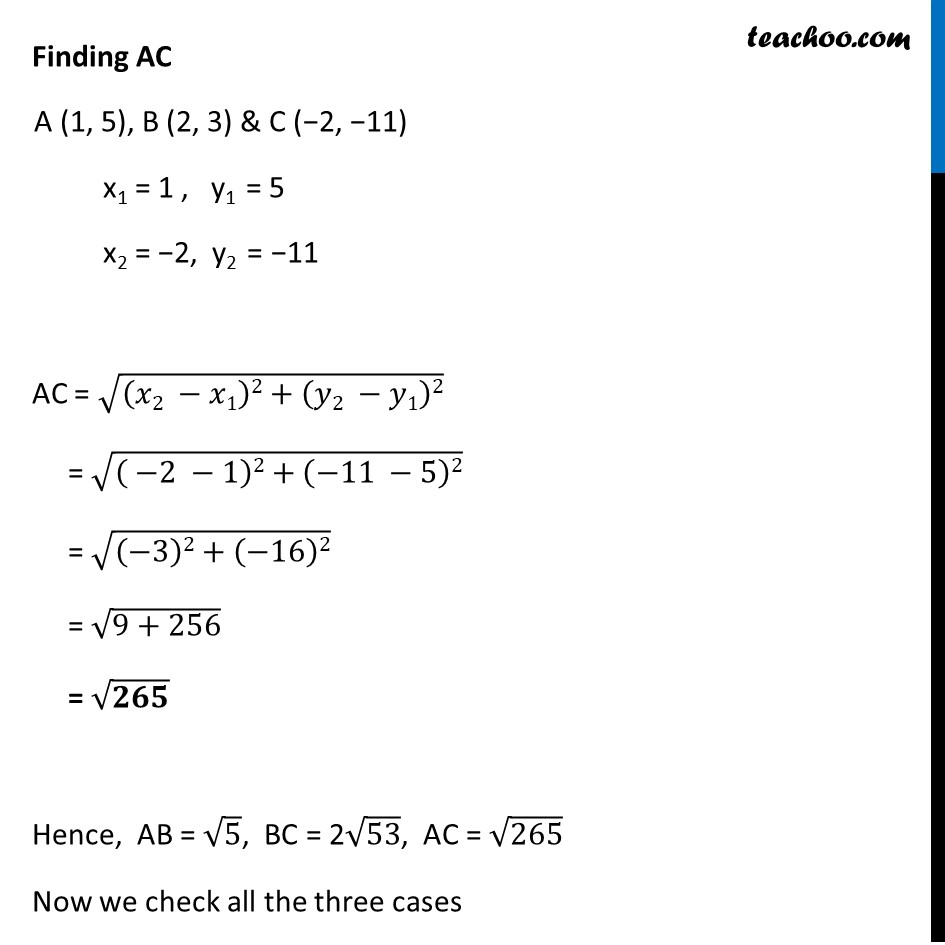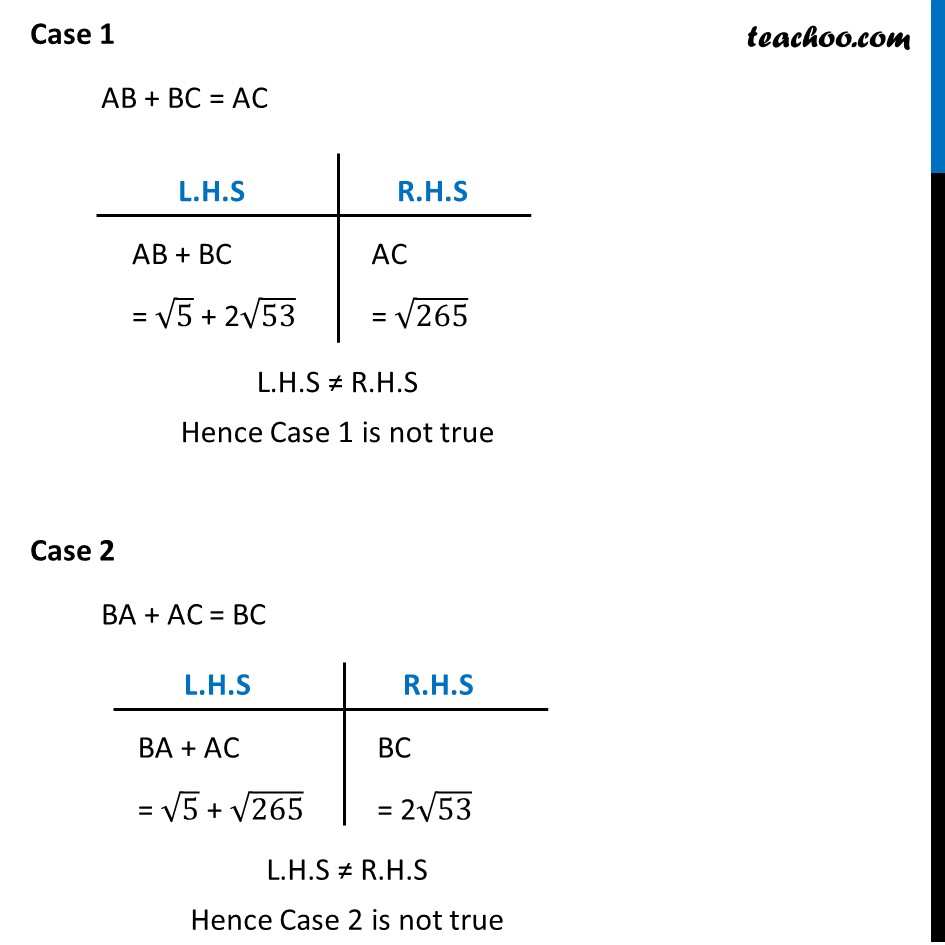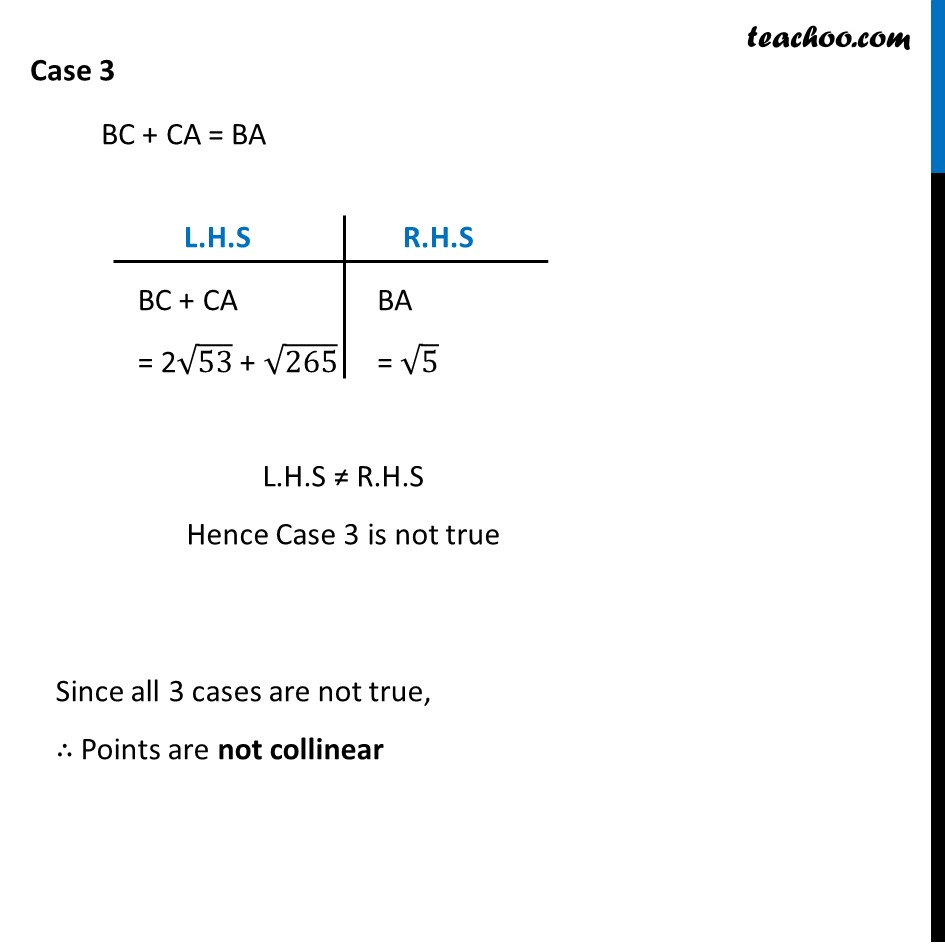Learn in your speed, with individual attention - Teachoo Maths 1-on-1 Class

### Transcript

Ex 7.1, 3 Determine if the points (1, 5), (2, 3) and (–2, – 11) are collinear. Let the 3 points be A (1, 5), B (2, 3) & C (−2, −11) Collinear points are points which fall on the same line There are three cases possible Case 1 A, B & C are collinear if AB + BC = AC Case 2 A, B & C are collinear if BA + AC = BC Case 3 A, B & C are collinear if BC + CA = BA Finding AB A (1, 5) & B (2, 3) x1 = 1, y1 = 5 x2 = 2, y2 = 3 AB = √((𝑥2 −𝑥1)2+(𝑦2 −𝑦1)2) = √(( 2 −1)2+(3 −5)2) = √((1)2+(−2)2) = √(1+4) = √𝟓 Finding BC B (2, 3) & C (−2, −11) x1 = 2, y1 = 3 x2 = −2, y2 = −11 BC = √((𝑥2 −𝑥1)2+(𝑦2 −𝑦1)2) = √(( −2 −2)2+(−11 −3)2) = √((−4)2+(−14)2) = √(16+196) = √212 = √(2×2×53) = 2√𝟓𝟑 Finding AC A (1, 5), B (2, 3) & C (−2, −11) x1 = 1 , y1 = 5 x2 = −2, y2 = −11 AC = √((𝑥2 −𝑥1)2+(𝑦2 −𝑦1)2) = √(( −2 −1)2+(−11 −5)2) = √((−3)2+(−16)2) = √(9+256) = √𝟐𝟔𝟓 Hence, AB = √5, BC = 2√53, AC = √265 Now we check all the three cases Case 1 AB + BC = AC L.H.S ≠ R.H.S Hence Case 1 is not true Case 2 BA + AC = BC Case 3 BC + CA = BA Since all 3 cases are not true, ∴ Points are not collinear